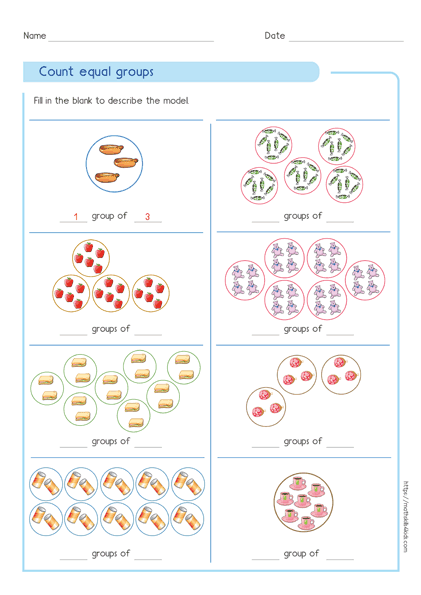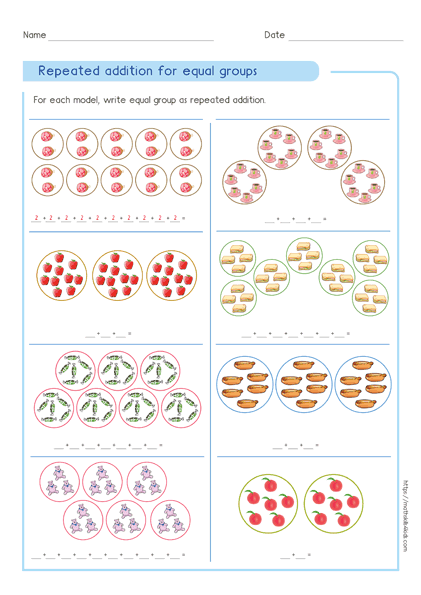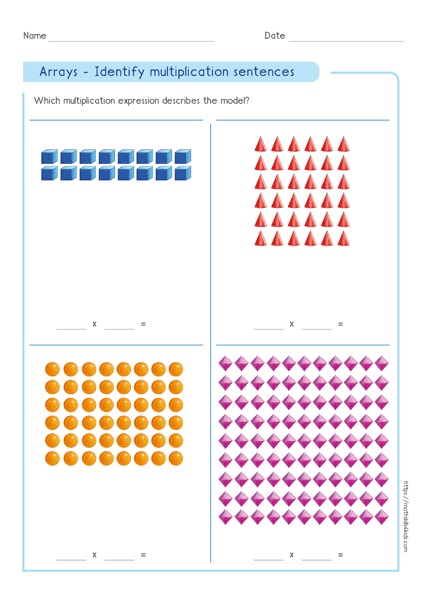# Free multiplication worksheets printable pdf and games

If you're a teacher or a parent looking for ways to help your child master multiplication, our free multiplication worksheets and games can be a great resource. Designed to cover the basic multiplication facts from 1 to 12, these printable pdfs can be a fun and engaging way to help children practice their multiplication skills. Additionally, we offer a variety of times table games and other fun multiplication practice activities to provide even more resources for teachers and students alike.

The best part is that these worksheets include multiplication practice for everyday situations and are completely free and easy to download. All you need to do is click on the link below the worksheets, and you will get access to a PDF file that contains all worksheets and answers.

## Multiplication practice for everyday situations

• Multiplication is a very useful skill that can help you in many everyday situations. For example, if you want to buy 12 apples and each apple costs 2 dollars, you can use multiplication to find out how much money you need. You just multiply 12 by 2 and get 24. That means you need 24 dollars to buy 12 apples. Easy, right?

Another example is if you want to share a pizza with your 3 friends. You also want to cut the pizza such each person eats 2 parts. Use multiplication to find out how many slices the pizza must be cut into. To get it, multiply 4 by 2, which is equal to 8. That means the pizza must have 8 slices for each person to get 2 slices of pizza. Yummy!

You can practice multiplication with any numbers you want. The more you practice, the faster and more confident you will become. Multiplication is fun and helpful, so don't be afraid to try it out!

### 1. UNDERSTANDING THE CONCEPT OF MULTIPLICATION

• Here are some tips and tricks on how to master math skills by understanding the concept of multiplication. Good!

So, let’s talk about one of the most important concepts in math: multiplication. Multiplication is a way of adding equal groups of objects or numbers together. It can help you solve problems faster and easier than just using addition. In this post, I win the following content, we will show you how to:

• Count equal groups.
• Identify multiplication expressions for equal groups.
• Write multiplication sentences for equal groups.
• Use repeated addition and multiplication for equal groups.
• Understand the expression for array.
• Write multiplication expressions for arrays.

### Some worksheets samples

•Print it...
•Print it...
•Print it...

### More skills for understanding multiplication with worksheets, online quizzes & tests

Have you downloaded this first set of worksheets to understand the concept of multiplication?
Great! Let's move on to the next level!

• ## 3. TIMES TABLES PRACTICE

Multiplication skill builders is made of excellent training games. It provides easy to use interactive interface for student to learn multiplication basics.

By using these contents, children from anywhere in the world will practicing and learning multiplication the easiest way.

## 5. ALL GRADES MULTIPLICATION RESOURCES

### WHAT TO LEARN ABOUT OUR MULTIPLICATION RESOURCES

Our multiplication times table exercises, training games & worksheets will bring to the excitement of kids, multiplication problems to be solved with pictures and visual models.

### Different approaches for a better understanding of multiplication

Using different approaches and strategies to solve multiplication problems is the key to easy understanding.
Our multiplication Worksheets & times table games however possesses four different strategies, namely repeated addition, array repartition, equal groups and number line.

These approaches are extremely very easy to understand.

Using these different approaches therefore, a kid will not only think about what works in multiplication, but also about how and why things work.

In view of all what has been said, our multiplication worksheets & Times Table Games has original elements for teaching and the best Multiplication Free Printable Resources.

•